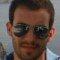Home » Core Java » Java Factorial ProgramNikos has graduated from the Department of Informatics and Telecommunications of The National and Kapodistrian University of Athens. During his studies he discovered his interests about software development and he has successfully completed numerous assignments in a variety of fields. Currently, his main interests are system’s security, parallel systems, artificial intelligence, operating systems, system programming, telecommunications, web applications, human – machine interaction and mobile development.

# Java Factorial Program

In this example we are going to talk about a classic programming task, as we are going to create a Java program that computes the factorial of a non negative integer. Those of you who know your math, you should already know:

The factorial of a positive integer `n`, annotated `n!`, is the product of all positive integers from 1 to n. Also, 0! = 1

Hence `n! = 1*2*3*4*5.....*n`. And so `4! = 1*2*3*4 = 24`. Pretty simple.

## 1. A simple for-loop calculation

This is the most straight forward implementation you can do to calculate the factorial of a non negative integer.

MathUtils.java:

 010203040506070809101112 `package` `com.javacodegeeks.core.factorial;` `public` `class` `MathUtils {` `    ``public` `static` `int` `simpleFactorial(``int` `n){``        ``int` `result = ``1``;``        ``for``(``int` `i = ``1``; i <= n; i++){``            ``result *= i;``        ``}``        ``return` `result;``    ``}``}`

This program is really self explanatory. We simply loop from 1 to n, adding the respectful number to the product at each iteration.

Let’s use this utility method in a simple program :

FactorialExample.java:

 1234567 `package` `com.javacodegeeks.core.factorial;` `public` `class` `FactorialExample {``    ``public` `static` `void` `main(String[] args){ ``        ``System.out.println(MathUtils.simpleFactorial(``10``));``    ``}``}`

If you run the program, this is the output:

 1 `3628800`

## 2. A recursive solution

There is no particular reason why you shouldn’t use the above simple for-loop implementation. In fact it’s the fastest one of those listed in this post. The reason is simply because it doesn’t use the call stack to hold the intermediate products, as recursive solutions do. But for the shake of completeness, we will provide recursive solutions as well. Having said that, you might find the following implementations handy in interview ventures.

MathUtils.java:

 010203040506070809101112 `package` `com.javacodegeeks.core.factorial;` `public` `class` `MathUtils {``    ` `    ``public` `static` `int` `recurciveFact(``int` `n){``        ``if``(n == ``0``){``            ``return` `1``;``        ``}``else``{``            ``return` `n*recurciveFact(n-``1``);``        ``}``    ``}``}`

Let’s break down the above method. Let’s, for example calculate `4!`.

 123456789 `recurciveFact(4) = 4*recurciveFact(3) ``    ``recurciveFact(3) = 3*recurciveFact(2) ``        ``recurciveFact(2) = 2*recurciveFact(1) ` `              ``recurciveFact(1) = 1*recurciveFact(0) =1*1 =1``                             ` `         ``recurciveFact(2) = 2*recurciveFact(1) = 2*1 = 2 ``    ``recurciveFact(3) = 3*recurciveFact(2) = 3*2 = 6 ``recurciveFact(4) = 4*recurciveFact(3) = 4*6 = 24 `

This is how recursion works in this case. So as you can see the intermediate example are calculated an stored in the call stack.

## 3. A tail-recursive solution

Tail recursion is a technique dictating that : There is nothing to do after the function returns except return its value.

MathUtils.java:

 01020304050607080910111213141516 `package` `com.javacodegeeks.core.factorial;` `public` `class` `MathUtils {` `    ``public` `static` `int` `tailrecurciveFact(``int` `n){``        ``return` `factorial(n,``1``);``    ``}``    ` `    ``private` `static` `int` `factorial(``int` `n,``int` `accum){``        ``if``(n==``0``)``            ``return` `accum;``        ``else``{``            ``return` `factorial(n-``1``,n*accum);``        ``}``    ``}``}`

Let’s break down the calculatation `4!`, so you can clearly see the case to support tail recursion.

 0102030405060708091011 `tailrecurciveFact(4) = factorial(4,1)``   ``factorial(4,1) =  factorial(3,4*1)``      ``factorial(3,4*1) =  factorial(2,4*1*3)``          ``factorial(2,4*1*) =  factorial(1,4*1*3*2)``  ` `             ``factorial(1,4*1*3*2) =  factorial(0,4*1*3*2*1) = 24` `          ``factorial(2,4*1*) = 24``      ``factorial(3,4*1) = 24``   ``factorial(4,1) = 24``tailrecurciveFact(4) = 24;`

As you can see the final result is already calculated as the algorithm descends in the call stack. So when the program returns, the result is already calculated and no more calculations are performed. Using this method, one can easily implement optimizations for what is the major problem with recursion: space . For example, one could use only the deeper stack frame to hold the result and immediately return the result of `tailrecurciveFact(4)`.

Several compilers implement different straightforward optimizations by flattening the tail recursion to a simple while loop. First you can imagine that the program could be transformed in the following pseudo-code (as goto is not implemented in Java):

 01020304050607080910 `private` `static` `int` `factorialGoto(``int` `n,``int` `accum){``    ``loop:``    ``if``(n==``0``)``        ``return` `accum;``    ``else``{``        ``accum*=n;``        ``n -= ``1``;``        ``goto` `loop;``    ``}``}`

It’s trivial to flatten this to a single while loop :

 1234567 `private` `static` `int` `factorialWhile(``int` `n,``int` `accum){``    ``while` `(n != ``0``) {``        ``accum *= n;``        ``n -= ``1``;``    ``}``    ``return` `accum;``}`

So you can see how tail recursion can be used to help the compiler produce really fast code, and on the same time you can keep using recursion, if you are having fun with functional programming. It is important to note though, that not all recursive programs can be transformed to tail recursive.

This was an example about factorial Program in Java.

You can download the source code of this example here: Java Factorial Program

Last updated on Sept. 13, 2019(No Ratings Yet)
Start the discussion Views Tweet it!

# Do you want to know how to develop your skillset to become a Java Rockstar?

## Subscribe to our newsletter to start Rocking right now!

### and many more ....